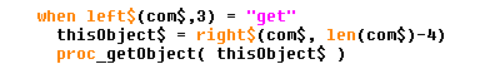# Writing an Adventure Game 06

Continuing the programming tutorial on building a simple adventure game, we will now look at allowing the player to pick up the objects we put into the game last time.

We shall keep the parsing of the language simple here for brevity.  First let's allow the player to type a command such as GET <OBJECT NAME>.  This is dealt with by the PROC_executeCommand() procedure as shown in the image:Adding the 'get

In pseudocode it works as follows:

WHEN THE FIRST THREE LETTERS OF THE COMMAND IS "GET"
PASS THE OTHER SIDE OF THE STRING TO PROC_getObject()

For example if the player types:

GET LAMP

the string "LAMP" is passed to the PROC_getLamp() procedure shown in detail below.Dealing with getting a 'thing'.

This procedure is quite complicated, and we will not deal with much more complicated than this in our tutorial.  It works by first checking whether the thing that the user has typed in is an object that exists in the game.  It then checks whether the player is at the same location as the object.  If they are then it adds the object to an array of objects currently carried by the player.

In pseudocode it works as follows:

LOOK THROUGH EACH OF THE OBJECTS IN THE GAME
IF THE THING THAT THE PLAYER TYPED IN MATCHES A VALID OBJECT
FOUND OBJECT

IF FOUND OBJECT
IF PLAYER AT SAME LOCATION AS OBJECT
LET PLAYER TAKE OBJECT
REMOVE OBJECT FROM THE WORLD
ELSE
TELL PLAYER THAT THERE ISN'T ONE HERE
ELSE
TELL PLAYER THAT THERE ISNT ONE HERE.

By the way, the first part of this algorithm is called a linear search.

Next time, we will look at objects that can be dropped, and interacting with objects in other ways.  For example.  I want to be able to eat that apple!

Source code follows:

REM an adventure game in BB4W
REM by Mr Street

REM version 1.0.0.1 setting up the world
REM version 1.0.0.2 moving around the world
REM version 1.0.0.3 fixing it so you can't walk out of the grid
REM version 1.0.0.4 health decreases each turn (because you get hungry)
REM version 1.0.0.5 set-up some objects for the player to interact with
REM version 1.0.0.6 allowing objects to be picked up

REM let's set up a 'world'

SIZE% = 3
DIM World\$( SIZE%, SIZE% ) : REM creates a 3x3 grid
REM now 'populate' the grid with some space names

PROC_populateWorld
REM now some data about our player
REM player's starting position

x% = 2
y% = 2
REM player's health

health% = 50

REM the objects

numObjects% = 2 : REM start with just a small number of objects

DIM prefix\$( numObjects% )   : REM eg "a" or "an" or "the"

DIM object\$( numObjects% )   : REM object name, eg "sword"

DIM objectx%( numObjects% )  : REM object x position

DIM objecty%( numObjects% )  : REM object y position

PROC_createObjects
REM a list of objects that the player has

DIM playerObject%( numObjects% )
PROC_main
STOP

DEFPROC_main
LOCAL command\$ : REM user's input
REM the main program
REM repeat until the player is dead

REPEAT

REM show the player's current position

PROC_showCurrent
REM show objects here

PROC_showObjects
REM get some input from the player

PRINT REM a blank line

INPUT "What now? > " command\$
REM deal with user's input

PROC_executeCommand( command\$ )
UNTIL health% <= 0
REM game over message

PRINT "GAME OVER!"
ENDPROC

DEFPROC_executeCommand( command\$ )
REM deals with the users input

LOCAL com\$, gridError\$
LOCAL ok% : REM checks whether the user's input is acceptable

ok% = TRUE REM assume user's input makes sense

gridError\$ = "You can't go in that direction!" : REM a message for when you move off the grid

com\$ = FN_convlc( command\$ ) : REM convert to lowercase so it is easier

CASE TRUE OF
WHEN
com\$ = "n" OR com\$ = "north"
IF y%<SIZE% THEN

y% += 1
ELSE
PRINT
gridError\$
ok% = FALSE
ENDIF

WHEN
com\$ = "s" OR com\$ = "south"
IF y% >1 THEN

y% -= 1
ELSE
PRINT
gridError\$
ok% = FALSE
ENDIF

WHEN
com\$ = "e" OR com\$ = "east"
IF x%<SIZE% THEN

x% += 1
ELSE
PRINT
gridError\$
ok% = FALSE
ENDIF

WHEN
com\$ = "w" OR com\$ = "west"
IF x%>1 THEN

x% -= 1
ELSE
PRINT
gridError\$
ok% = FALSE
ENDIF

WHEN LEFT\$(
com\$,3) = "get"
thisObject\$ = RIGHT\$(com\$, LEN(com\$)-4)
PROC_getObject( thisObject\$ )

OTHERWISE
PRINT
"I don't understand you!"
ok% = FALSE
ENDCASE

REM if the user's input was acceptable, then they have
REM made one 'turn' and should lose one health point

IF ok% THEN

health% -= 1
ENDIF

ENDPROC

DEFPROC_showCurrent
PRINT "You are at the : "World\$(x%, y%)
PRINT "Your health is : "STR\$(health%)
ENDPROC

DEFPROC_showObjects
REM look for any objects here

LOCAL n%
REM look through list of objects

FOR n% = 1 TO numObjects%
REM is the player's location the same as the object location?

IF x% = objectx%(n%) AND y% = objecty%(n%) THEN

REM objects is here

PRINT "There is "prefix\$(n%)" "object\$(n%)" here."
ENDIF
NEXT
ENDPROC

DEFPROC_getObject( this\$ )
REM allows player to pick up objects

LOCAL n%, found%, index%
LOCAL objectError\$ : objectError\$ = "I don't see one of those here!"
WHILE n% <=numObjects% AND NOT found%
IF this\$ = object\$(n%) THEN

found% = TRUE

index% = n%
ELSE

n% += 1
ENDIF
ENDWHILE

:
IF found% THEN

REM check that the object is at the player's location

IF x% = objectx%(index%) AND y% = objecty%(index%) THEN

REM object is here, so pick it up

playerObject%(index%) = TRUE REM add to list of player's objects

objectx%(index%) = -1 : REM remove object from the grid

objecty%(index%) = -1 : REM remove object from the grid

PRINT "OK, you take "prefix\$(index%)" "object\$(index%)
ELSE
PRINT
objectError\$
ENDIF
ELSE
PRINT
objectError\$
ENDIF
ENDPROC

DEF FN_convlc(A\$)
REM converts to lower case

SYS "CharLowerBuff", !^A\$, LEN(A\$)
= A\$

DEFPROC_populateWorld
REM puts some names of the spaces into the World

LOCAL x%, y%

FOR y% = 1 TO SIZE%
FOR x% = 1 TO SIZE%
NEXT
NEXT
ENDPROC

:
REM the data for our 3x3 grid

DATA "Hills",  "Mountains", "Forest"

DATA "Castle", "Village", "Fields"

DATA "Woods",  "Swamp",  "Lake"

DEFPROC_createObjects
REM puts the names of objects into a list

LOCAL n%
FOR n% = 1 TO numObjects%